## Introduction

Refraction in classic optics was recently re-visited from the viewpoints of complex refractive index of a bulky medium1, abrupt phase change of an interface2 and diffraction theory for gratings3. Furthermore, these works shed light on the relation between the reflection and incidence, interpreted as the generalized Snell's law of reflection (GSL)2, a novel way to optical wavefront engineering, resulting in promising accomplishments4,5,6,7,8. In optics, the phase-inhomogeneous metasurfaces realized by thin metallic nanoantennas conserve the wave number along an interface while impose the extra phase accumulation2. Fundamental physics is explained by phased antenna array9,10,11.

In principle, GSL is based on Fermat's principle, which holds for all monochromatic waves. However, the luxury of using metallic metasurfaces2,4 to fulfill the optical phase control is no more available in acoustics due to the limited choice of acoustic materials. Thus, the variable in GSL: phase change on a flat surface becomes an abstract concept in acoustics without any design principle and practical clue. Therefore, it is indispensable and valuable to establish a different principle to manipulate acoustic waves.

In this paper, we establish the framework of acoustic wavefront manipulation by resorting to the specific acoustic impedance (SAI)12 inhomogeneity and discontinuity, rather than phase inhomogeneity in terms of wave propagation1,2. SAI is one of the acoustic properties of a material, more possible to be controllable in reality than that propagation phase. More specifically, we find out the inhomogeneous SAI will generally give rise to one ordinary reflection pro and one extraordinary reflection pre, i.e., double reflections. Furthermore, the flat inhomogeneous SAI surface is able to switch on or off pro without the influence on its direction, but tweak pre in the manner of our proposed design principle: impedance-governed generalized Snell's law of reflection (IGSL).

## Results

### Theory: steerable extraordinary reflection and switchable ordinary reflection

The inhomogeneous SAI Zn of the flat surface can be expressed as a complex, whose real and imaginary parts may change spatially. In order to reduce the complexity of modeling as the beginning attempt, we set the real part as a spatial constant. Later we prove that the spatial varying of the real part cannot support our results, which is derived in detail in Supplementary Information. We consider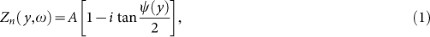where A is an arbitrary constant irrelevant to any spatial change and ψ(y) is the variable for the imaginary part. Note that ω-dependency on the right hand side of Eq. (1) has already been included in ψ(y). The total acoustic pressure p in the upper space satisfies the integral equation: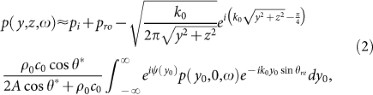where pi denotes the incidence; ρ0 and c0 are the density and the speed of sound in the upper space in Fig. 1(a); k0 = ω/c0 is the wave number; θ* is constant; θre is the angle of pre. According to Supplementary Information, both pro and pre exist for a general A, implying the unusual double reflections:After applying the first-order approximation and the stationary phase approximation to Eq. (4), the relation between θre and the incident angle θi is unveiled:Note that based on our derivation, only when the inhomogeneous SAI along the flat surface is expressed in form of Eq. (1) can our IGSL survive. Although IGSL's appearance is similar to GSL1,2,4, its physical meaning of ψ(y) are dramatically different. Fundamentally, the variable of our IGSL Eq. (5) is about the value of surface acoustic impedance instead of the abrupt propagating phase change. Moreover, IGSL only serves to steer pre at will, with no influence on the direction of pro, as illustrated in Fig. 1(a). In Supplementary Information we highlight the irrelevance between GSL and our proposed IGSL. In addition, GSL mentions the extra accumulated phases along wave-propagation paths, but it is still relying on graphical methods to find out the relation between the configuration of the passive antenna array and the needed phase in optics2. However, we do not have the passive antenna in acoustics. Here, IGSL Eq. (5) and Eq. (1), serving as an explicit design rule, provide us the feasible way based on a different mechanism in acoustics.

Eq. (5) also sheds the light on an extreme angle (similar to critical angle):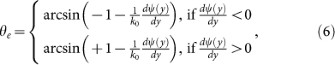above which pre becomes evanescent in the upper space. Eq. (6) holds only when. Otherwise, pre becomes evanescent.

Usually, both pro and pre will coexist as shown in Fig. 1(a), suggesting double reflections, while IGSL only controls θre. Hence, it is interesting to eliminate pro as shown in Fig. 1(b), by means of a particularly selected value of A. Eq. (3) suggests that A = (ρ0c0)/(2 cos θi) can make pro vanish, i.e., pro is switched off, as shown in Fig. 1(b). The corresponding SAI of the flat surface then becomes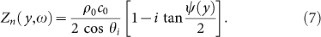### Verification: continuous impedance and discontinuous impedance

Supposing the gradient of ψ(y) along the flat interface is constant, we notice Eq. (4) turns out to be a Dirac Delta without approximation. From Eq. (5) we predict the wavefront of pre will propagate in the form of a plane acoustic wave, independent of y. We select water (ρ0 = 1 kg/m3; c0 = 1500 m/s12) as the background medium, ω = 300 K rad/s as the circular frequency,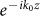as the normal incident plane ultrasound and a linear form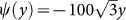in Eq. (7).

θre is theoretically found to be −60° by IGSL, validated by our simulation in Fig. 1(d). pro is thoroughly suppressed thanks to the specific A chosen according to Eq.(1). In contrast, in Fig. 1(c), the same parameters are kept except for another A, whose value is arbitrarily taken to be ρ0c0. It clearly shows that pro occurs and interferes with pre, but pre still keeps the same, verifying our theoretical formulation. In terms of phenomena, the designed inhomogeneous SAI Eq. (1) essentially implies the changes of both the propagating phases and amplitudes, only by which the effect of double reflections may occur. In terms of physics, the extra momentum supplied by the metasurface is employed to compensate the momentum mismatch between the incident acoustic beams and the diffracted beams. Therefore, for the double backward propagating beams, pro is the most pervasive specular reflection, while pre is attributed to the diffraction of higher order.

Fig. 1(d) suggests the possibility of negative reflection for pre, which is further verified for oblique incidence in Fig. 2. In Fig. 2(a), because of the inhomogeneous SAI and the arbitrary A in Eq. (1), both pro and pre occur. Fig. 2(b) depicts the same situation except for pro being switched off as a result of the specifically chosen A according to Eq. (7), while the red line pre stays the same as that in Fig. 2(a). The blue braces represent the region of negative pre. It is noteworthy that pre does not exist if θi is beyond the extreme angle θe = −30° in Eq. (6), corresponding to the purple dots. One field simulation is provided in Supplementary Information.

As depicted in Fig. 1(g), we propose one plausible realization schematic for the general SAI of Eq. (1), where all hard-sidewall tubes with one pressure-release termination are gathered and juxtaposed perpendicular to the flat interface. Observed at the top view, each tube has a square cross section whose width is d, with four enclosed hard sidewalls (black). Then observed at the side view, the upside open termination of each tube constitutes an effective SAI pixel of the interface, while the other end sealed by a thin film (orange) serves as the pressure-release termination12. The upper space and the interior of each tube are filled with water, without separation. The light blue indicates air downside, which is isolated from water by the thin film.

The SAI of each tube at the opening facing the upper space is12: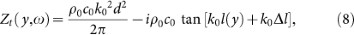where l(y) is the length of each tube and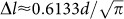is the effective end correction. By comparison of Eq. (1) and Eq. (8), it is required that A = ρ0c0k02d2/(2π) and A tan[ψ(y)/2] = ρ0c0 tan [k0l(y) + k0Δl], leading to the value of the spacing d for impedance discretization and the dependance between l(y) and ψ(y):where the arbitrary integer n is required to be set suitably to make l a positive value. Thus, the change of ψ along y, representing the control of pre, is interpreted as the change of l, implying one straightforward realization based on discontinuous impedance. Thus, the inhomogeneity of the acoustic impedance is strictly paraphrased into the inhomogeneity of the tube-array structure, resulting in our acoustic metasurface. At the side view in Fig. 1(g), the solid red contour indicates one arbitrary function of l(y) calculated from Eq. (9). Based on the discretization d calculated from Eq. (9) as well, we are able to find d and the corresponding height l(y), marked with the yellow dots. Note that the top of the tube array is aligned into a flat surface (red dashed line), above which acoustic waves impinge. Thus, the change of tube lengths will not affect the flatness of the surface. In addition, thanks to the property of the arc-tangent in Eq. (9), the tube-array metasurface is within a thin layer without the space-coiling-up technique13. It is also noteworthy that because of the intrinsic differences between optics and acoustics, so far we cannot obtain the mechanism-analog of the optical metasurface, which is based on resonances and independent with the thickness or effective propagating lengths, but we can achieve the phenomenon-analog in acoustics using the tube array. In principle, because tubes can be regarded as Helmholtz resonators, complex SAI at each pixel can be realized by a suitable arrangement of resonators, as the analog of the complex electric impedance realized by the combination of resistance, capacitance and inductance. In addition, we know that only the real part, the electric resistance, consumes energy while the imaginary part does not. In the same manner in acoustics, the energy loss is theoretically only attributed to the real part of the surface complex SAI in Eq. (8), i.e., the loss in our case is caused by the energy consumption from the tube array.

Using this method, we reproduce Fig. 1(c)(d) by realistic impedance discontinuity, so as to verify our proposed realization. In Fig. 1(e)(f), d = 0.0125 and 0.00886 are selected respectively according to Eq. (9) and the corresponding contours of the tube length l in terms of the location y are illustrated as the red lines, respectively. Fig. 1(e) shows strong interference between pre and pro while Fig. 1(f) shows the nearly undisturbed pre, coinciding with Fig. 1(c) and (d) respectively.

### Application: acoustic illusion and ipsilateral focusing

To demonstrate IGSL's capability of designing novel acoustic devices, we metamorphose acoustic pressure fields everywhere through SAI manipulation as simulated in Fig. 3. This deceptive effect is obtained by manipulating plane wavefronts into wavefronts generated by a virtual reflector or focusing illumination, governed by the control of pre, i.e., IGSL. Under these scenarios, we need to consider nonlinear forms of ψ(y). New phenomena are thus expected when θre becomes spatially varying.

It is found that the acoustic deception can be created via IGSL, e.g., ψ(y) = 0.7 y2 in Eq. (7), resulting in pro = 0. Correspondingly, θre in Fig. 3(a) is a position-dependent function sin θre = 0.14 y, in which case pre fans out as demonstrated in Fig. 3(a), verifying our theory. Here the spacing d for impedance discretization is 0.1772 and the relations between l and y derived from Eq.(9) are enclosed in Fig. 3. Therefore, IGSL can be employed to camouflage a flat surface as if there were a curvilinear object at the origin instead of the physical planar interface. The dual effect allowing a curved reflector to mimic a flat mirror, by manipulating the convex wavefronts into planar wavefronts, was reported in plasmonic regime19.

Furthermore, the SAI can be designed to make acoustic waves reflected by a planar interface focused as well. In optics, a flat lens with metallic nanoantennas of varying sizes and shapes can consequently converge the transmitted light to a focal point5,6. Note that the optical focusing controlled by optical GSL is on the other side of incoming lights, i.e., on two sides of the flat surface5,6 in the transmission mode. In acoustics, we employ an inhomogeneous SAI flat surface to focus pre, in the reflection mode by IGSL without pro.

This ipsilateral focusing in Fig. 3(b), is thus obtained in the planar geometry in acoustics for the first time. In Eq. (7), a hyperbolic form is set:(f being the given focal length20) for the SAI of the flat interface. pre from different angles constructively interferes at the ipsilateral focal point, as if the waves emerge from a parabolic surface. The parameters in Fig. 3(b) are the same as those in Fig. 3(a) except for the specific hyperbolic SAI form, with the designed focal point at (y = 0, z = 4) and pro suppressed. In addition, the simulated acoustic pressure by impedance discretization at the focal point is well confined at (y = 0, z = 4).

Interestingly, the imaging at the same side was previously presented for electromagnetic waves14,15. In14, it demands strong chiral materials filled in the whole upper space. The same-side imaging is only a partial imaging, i.e., only one circularly polarized wave being imaged and the other being reflected ordinarily. In acoustics, our ipsilateral imaging is achieved by translating all the stringent requirements of the half-space chiral materials into an inhomogeneous impedance surface. In electromagnetism, ipsilateral imaging can be achieved as well by surface gratings15 or antenna arrays. However, the polarization of incident electromagnetic waves is always closely related to the effect of focusing. Therefore, the ipsilateral imaging in acoustics by IGSL has no polarization constraints thanks to the acoustic wave nature, i.e., longitudinal vibration.

### Application: conversion from propagating acoustic waves to surface acoustic waves

Beyond the acoustic-field metamorphosis, we further establish a kind of acoustic cognitive deception about a SAI surface converting propagating acoustic waves (PAW) to surface acoustic waves (SAW) in Fig. 4, by means of IGSL. The extreme angle 0° in Eq.(6) demands ψ(y) = ± 10 y. Therefore, we set the SAI of Eq. (7) slightly over that extreme, e.g., ψ(y) = −11 y for y < 0 and ψ(y) = 11 y for y > 0 are set along the flat interface symmetrically with respect to the z. In Fig. 4(a), the bidirectional surface acoustic waves are attributed to the coupling effect governed by the diffracted evanescent pre which propagates along the metasurface16. Owing to the inhomogeneous SAI interface, the ideally perfect conversion comes true in acoustics except for a little diffraction. Physically, the SAI along the flat surface provides an extra momentum to compensate the momentum mismatch between propagating waves and surface waves in acoustics, resulting in the high efficiency conversion. In contrast, if one uses a constant SAI Eq.(7) with ψ(y) = 11 along the flat surface (the homogenous SAI does not generate pre; only pro occurs), the reflected sound pressure level in Fig. 4(c) is almost uniformly spread over the space.